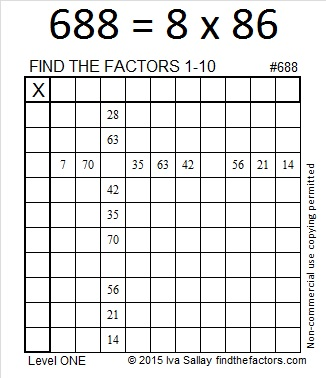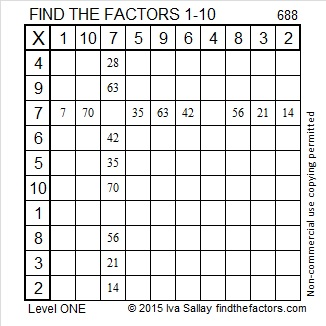# 688 is a Friedman number

688 is a palindrome in two different bases:

• 2002 in base 7; note that 2(343) + 0(49) + 0(7) + 2(1) = 688
• 494 in base 12; note that 4(144) + 9(12) + 4(1) = 688

688 is called a Friedman number because it can be expressed using only its own digits and +, -, x, ÷, ( ), and exponents. 688 = 8 x 86 so I made a factor tree based on that single multiplication fact:Since it’s such a fun number fact, I positioned it on top of today’s factoring puzzle, too.Print the puzzles or type the solution on this excel file: 10 Factors 2015-11-23

—————————————————————————————————

• 688 is a composite number.
• Prime factorization: 688 = 2 x 2 x 2 x 2 x 43, which can be written 688 = 2⁴ x 43
• The exponents in the prime factorization are 4 and 1. Adding one to each and multiplying we get (4 + 1)(1 + 1) = 5 x 2 = 10. Therefore 688 has exactly 10 factors.
• Factors of 688: 1, 2, 4, 8, 16, 43, 86, 172, 344, 688
• Factor pairs: 688 = 1 x 688, 2 x 344, 4 x 172, 8 x 86, or 16 x 43
• Taking the factor pair with the largest square number factor, we get √688 = (√16)(√43) = 4√43 ≈ 26.229754.—————————————————————————————————## 2 thoughts on “688 is a Friedman number”

1.Steve Morris

Gosh, I never realised how special each and every number truly is! According to Wikipedia, 14972 is the lowest number that is not interesting in some way. Paradoxically that makes it very interesting.

•ivasallay

The writer of Wikipedia just chose that number at random. (Archemedes Lab did the same thing with 684, but I found interesting properties of that number, too.)
Numberphile states that 14972 is the smallest number not to appear in any sequence in the On-line Encyclopedia of Integer Sequences, and then used the same paradoxical proof you mentioned to prove that 14972 is an interesting number.

2 x 2 x 19 x 197 = 14972. The fact that it can be factored is interesting. In fact, it has 12 factors. If it couldn’t be factored, that would be interesting too.
From its factors I know that its square root can be reduced, and it’s the hypotenuse of a Pythagorean triple.

I also know that it can be written as the sum of 8 consecutive numbers, the sum of 19 consecutive numbers, and as the sum of 152 consecutive numbers.

14972 is 5656 in BASE 14, M3M in BASE 26, and CWC in BASE 33.

This site uses Akismet to reduce spam. Learn how your comment data is processed.